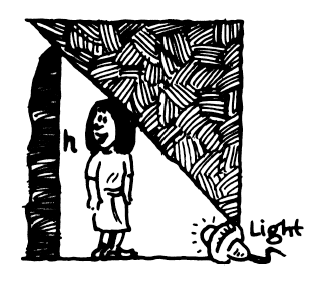### Home > PC3 > Chapter 6 > Lesson 6.1.3 > Problem6-52

6-52.A shadow of Kate cast on a wall by a light on the ground, which is $d$ meters from the wall. Kate is $162$ centimeters tall and is $3$ meters from the wall. Express $d$ as a function of the height of the shadow, $h$.

1. Label the drawing.
2. Convert Kate's height to meters.
3. You have two similar triangles. Write a proportion with $d$ and $h$.
4. Solve for $d$.

$\frac{d-3}{d}=\frac{1.62}{h}$